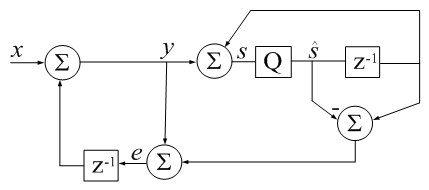# Accumulator Error Feedback

(Difference between revisions)
 Revision as of 21:04, 29 January 2018 (edit) (→sorting)← Previous diff Revision as of 21:04, 29 January 2018 (edit) (undo) (→sorting)Next diff → Line 43: Line 43: === sorting === === sorting === - Floating point compensated summation accuracy is data dependent. + Floating-point compensated summation accuracy is data dependent. Substituting a unit sine wave at arbitrary frequency, instead of a random number sequence input, Substituting a unit sine wave at arbitrary frequency, instead of a random number sequence input, can make compensated summation fail to produce more accurate results than a simple sum. can make compensated summation fail to produce more accurate results than a simple sum.

## Revision as of 21:04, 29 January 2018csum() in Digital Signal Processing terms: z-1 is a unit delay,
Q is a 64-bit floating-point quantizer. Algebra represents neither a sequence of instructions or algorithm. It is only meant to remind that an imperfect accumulator introduces noise into a series.
qi represents error due to quantization (additive by definition).
```function s_hat = csum(x)
% CSUM Sum of elements using a compensated summation algorithm.
%
% This Matlab code implements
% Kahan's compensated summation algorithm (1964)
% which takes about twice as long as sum() but
% produces more accurate sums when number of elements is large.
%   -David Gleich
%
% Also see SUM.
%
% Example:
% clear all; clc
% csumv=0;  rsumv=0;
% n = 100e6;
% t = ones(n,1);
% while csumv <= rsumv
%    v = randn(n,1);
%
%    rsumv = abs((t'*v - t'*v(end:-1:1))/sum(v));
%    disp(['rsumv = ' num2str(rsumv,'%1.16f')]);
%
%    csumv = abs((csum(v) - csum(v(end:-1:1)))/sum(v));
%    disp(['csumv = ' num2str(csumv,'%1.16e')]);
% end

s_hat=0; e=0;
for i=1:numel(x)
s_hat_old = s_hat;
y = x(i) + e;
s_hat = s_hat_old + y;
e = y - (s_hat - s_hat_old);
end
return
```

### sorting

Floating-point compensated summation accuracy is data dependent. Substituting a unit sine wave at arbitrary frequency, instead of a random number sequence input, can make compensated summation fail to produce more accurate results than a simple sum.

In practice, input sorting can sometimes achieve more accurate summation. Sorting became integral to later algorithms, such as those from Knuth and Priest. But the very same accuracy dependence on input data prevails.